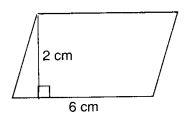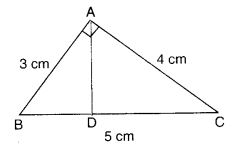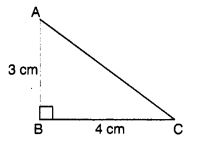Check the below Online Education NCERT MCQ Questions for Class 7 Maths Chapter 11 Perimeter and Area with Answers Pdf free download. MCQ Questions for Class 7 Maths with Answers were prepared based on the latest exam pattern. We have provided Perimeter and Area Class 7 Maths MCQs Questions with Answers to help students understand the concept very well. https://ncertmcq.com/mcq-questions-for-class-7-maths-with-answers/

Students can also refer to NCERT Solutions for Class 7 Maths Chapter 11 Perimeter and Area for better exam preparation and score more marks.

## Online Education for Perimeter and Area Class 7 MCQs Questions with Answers

Perimeter And Area Class 7 MCQ Question 1.
Perimeter of a square =
(a) side × side
(b) 3 × side
(c) 4 × side
(d) 2 × side

Hint:
Formula

Class 7 Maths Chapter 11 MCQ Question 2.
Perimeter of a rectangle of length Z and breadth 6 is
(a) l + b
(b) 2 × (l + b)
(c) 3 × (l + b)
(d) l × b

Answer: (b) 2 × (l + b)
Hint:
Formula

MCQ Of Perimeter And Area Class 7 Question 3.
Area of a square =
(a) side × side
(b) 2 × side
(c) 3 × side
(d) 4 × side

Hint:
Formula

MCQ On Perimeter And Area For Class 7 Question 4.
Area of a rectangle of length l and breadth b is
(a) l × b
(b) l + b
(c) 2 × (l + b)
(d) 6 × (l + b)

Hint:
Formula

MCQ Questions On Area And Perimeter For Class 7 Question 5.
Area of a parallelogram =
(а) base × height
(b) $$\frac { 1 }{ 2 }$$ × base × height
(c) $$\frac { 1 }{ 3 }$$ × base × height
(d) $$\frac { 1 }{ 4 }$$ × base × height

Hint:
Formula

Class 7 Maths Chapter 11 MCQ With Answers Question 6.
Area of a triangle =
(а) base × height
(b) $$\frac { 1 }{ 2 }$$ × base × height
(c) $$\frac { 1 }{ 3 }$$ × base × height
(d) $$\frac { 1 }{ 4 }$$ × base × height

Answer: (b) $$\frac { 1 }{ 2 }$$ × base × height
Hint:
Formula

Class 7 Maths Ch 11 MCQ Question 7.
The circumference of a circle of radius r is
(a) πr
(b) 2πr
(c) πr2
(d) $$\frac { 1 }{ 4 }$$ πr2

Hint:
Formula

Class 7 Perimeter And Area MCQ Question 8.
The circumference of a circle of diameter d is
(a) πd
(b) 2πd
(c) $$\frac { 1 }{ 2 }$$ πd
(d) πd2

Hint:
Formula

Perimeter And Area MCQ Class 7 Question 9.
If r and d are the radius and diameter of a circle respectively, then
(a) d = 2 r
(b) d = r
(C) d = $$\frac { 1 }{ 2 }$$ r
(d) d = r2

Answer: (a) d = 2 r
Hint:
Formula

Class 7 Maths Chapter 11 Extra Questions Question 10.
The area of a circle of radius r is
(a) πr2
(b) 2πr2
(c) 2πr
(d) 4πr2

Hint:
Formula

MCQ On Area And Perimeter Class 7 Question 11.
The area of a circle of diameter d is
(a) πd2
(b) 2πd2
(c) $$\frac { 1 }{ 4 }$$ πd2
(d) 2πd

Answer: (c) $$\frac { 1 }{ 4 }$$ πd2
Hint:
Formula

Perimeter And Area Class 7 Extra Questions Question 12.
1 cm2 =
(a) 10 mm2
(b) 100 mm2
(c) 1000 mm2
(d) 10000 mm2

Hint:
Formula

Class 7 Math Chapter 11 MCQ Question 13.
1 m2 =
(a) 10 cm2
(b) 100 cm2
(c) 1000 cm2
(d) 10000 cm2

Hint:
Formula

Class 7 Perimeter And Area Extra Questions Question 14.
1 hectare =
(a) 10 m2
(b) 100 m2
(c) 1000 m2
(d) 10000 m2

Hint:
Formula

MCQ Questions For Class 7 Maths Chapter 11 Question 15.
1 are =
(a) 10 m2
(b) 100 m2
(c) 1000 m2
(d) 10000 m2

Hint:
Formula

Question 16.
The area of a square plot is 1600 m2. The side of the plot is
(a) 40 m
(b) 80 m
(c) 120 m
(d) 160 m

Hint:
Side = $$\sqrt{1600}$$ = 40 m

Question 17.
The area of a square is 121 m2. Its side is
(a) 11 m
(b) 21 m
(c) 31 m
(d) 41 m

Hint:
Side = $$\sqrt{121}$$ = 11 m

Question 18.
The area of a square is 625 m2. Find its side
(a) 25 m
(b) 50 m
(c) 125 m
(d) 5 m

Hint:
Side = $$\sqrt{625}$$ = 25 m

Question 19.
The area of a rectangular field is 250 m2. If the breadth of the field is 10 m, find its length.
(a) 25 m
(b) 50 m
(c) 100 m
(d) 125 m

Hint:
Length = $$\frac{250}{10}$$ = 25 m

Question 20.
The perimeter of a rectangle is 30 m. Its length is 10 m. Its breadth is
(a) 5 m
(b) 10 m
(c) 15 m
(d) 3 m

Hint:
30 = 2 (Length + Breadth)
⇒ 30 = 2 (10 + Breadth)

Question 21.
The perimeter of a square is 48 cm. Its area is
(a) 144 cm2
(b) 12 cm2
(c) 48 cm2
(d) 100 cm2

Hint:
Side = $$\frac{48}{4}$$ = 12 cm
∴ Area = 12 × 12 = 144 cm2

Question 22.
The area of a rectangular room is 150 m2. If its breadth is 10 m, then find its length.
(a) 15 m
(b) 25 m
(c) 50 m
(d) 55 m

Hint:
Length = $$\frac{150}{10}$$ = 15 m

Question 23.
A rectangular wire of length 40 cm and breadth 20 cm is bent in the shape of a square. The side of the square is
(a) 10 cm
(b) 20 cm
(c) 30 cm
(d) 40 cm

Hint:
Side of square = $$\frac{2(40+20)}{4}$$ = 30 cm

Question 24.
The area of a parallelogram of base 5 cm and height 3.2 cm is
(a) 8 cm2
(b) 12 cm2
(c) 16 cm2
(d) 20 cm2

Hint:
Area = 5 × 3.2 = 16 cm2

Question 25.
Find the area of the following ; parallelogram:(a) 12 cm2
(b) 6 cm2
(c) 24 cm2
(d) 8 cm2

Hint:
Area = 6 × 2 = 12 cm2

Question 26.
Find the area of the following parallelogram:(a) 6 cm2
(b) 12 cm2
(c) 16 cm2
(d) 9 cm2

Hint:
Area = 4 × 3 = 12 cm2

Question 27.
The area of the parallelogram ABCD in which AB = 6.2 cm and the perpendicular from C on AB is 5 cm is
(a) 30 cm2
(b) 29 cm2
(c) 28 cm2
(d) 31 cm2

Hint:
Area = 6.2 × 5 = 31 cm2

Question 28.
One of the sides and the corresponding height of a parallelogram are 3 cm and 1 cm respectively. The area of the parallelogram is
(a) 1 cm2
(b) 3 cm2
(c) 6cm2
(d) 12 cm2

Hint:
Area = 3 × 1 = 3 cm2

Question 29.
If the area of a parallelogram is 16 cm2 and base is 8 cm, find the height.
(a) 1 cm
(b) 2 cm
(c) 3 cm
(d) 4 cm

Hint:
Height = $$\frac{16}{8}$$ = 2 cm

Question 30.
The area of a parallelogram is 20 cm2 and height is 2 cm. Find the corresponding base.
(a) 3 cm
(b) 4 cm
(c) 5 cm
(d) 2.4 cm

Hint:
Base = $$\frac{20}{2}$$ = 10 cm

Question 31.
Find AD in the following figure :(a) 3 cm
(b) 4 cm
(c) 5 cm
(d) 2.4 cm

Hint:
Area of Δ ABC = $$\frac{3×4}{2}$$
= $$\frac{5×AD}{2}$$ ⇒ AD = 2.4

Question 32.
Find the area of ∆ ABC :(a) 1 cm2
(b) 2 cm2
(c) 4 cm2
(d) $$\frac { 1 }{ 2 }$$ cm2

Answer: (d) $$\frac { 1 }{ 2 }$$ cm2
Hint:
Area = $$\frac{1×1}{2}$$ = $$\frac{1}{2}$$ cm2

Question 33.
Find the area of ∆ ABC(a) 3 cm2
(b) 4 cm2
(c) 6 cm2
(d) 12 cm2

Hint:
Area = $$\frac{4×3}{2}$$ = 6 cm2

Question 34.
Find the area of ∆ ABC(a) 1 cm2
(b) 2 cm2
(c) 3 cm2
(d) 4 cm2

Hint:
Area = $$\frac{3×2}{2}$$ = 3 cm2

Question 35.
Which of the following is not the value of π ?
(a) $$\frac { 22 }{ 7 }$$
(b) $$\frac { 7 }{ 22 }$$
(c) $$\frac { 355 }{ 113 }$$
(d) 3.14

Answer: (b) $$\frac { 7 }{ 22 }$$

Question 36.
The radius of a circle is 7 cm. Its circumference is
(a) 22 cm
(b) 44 cm
(c) 11 cm
(d) 66cm

Hint:
Circumference = 2 × $$\frac{22}{7}$$ × 7 = 44 cm

Question 37.
The diameter of a circle is 14 cm. Find its circumference
(a) 44 cm
(b) 22 cm
(c) 11 cm
(d) 55 cm

Hint:
Circumference = $$\frac{22}{7}$$ × 14 = 44 cm

Question 38.
The radius of a circle is 7 cm. Find its area
(a) 154 cm2
(b) 77 cm2
(c) 11 cm2
(d) 22 cm2

Hint:
Area = $$\frac{22}{7}$$ × 7 × 7 = 154 cm2

Question 39.
The diameter of a circle is 7 cm. Find its area
(a) 154 cm2
(b) 38.5 cm2
(c) 22 cm2
(d) 11 cm2

Hint:
Area = $$\frac{22}{7}$$ × $$\frac{7}{2}$$ × $$\frac{7}{2}$$ = 38.5 cm2

Question 40.
The perimeter of the following figure is(a) 27 cm
(b) 28 cm
(c) 36 cm
(d) 40 cm

Perimeter = 2 × 7 + $$\frac{22}{7}$$ × 7